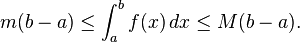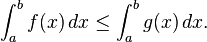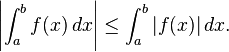# INEQUALITIES FOR INTEGRALS

A number of general inequalities hold for Riemann-integrable functions defined on a closed and bounded interval [a, b] and can be generalized to other notions of integral (Lebesgue and Daniell).

• Upper and lower bounds. An integrable function f on [a, b], is necessarily bounded on that interval. Thus there are real numbers m and M so that mf (x) ≤ M for all x in [a, b]. Since the lower and upper sums of f over [a, b] are therefore bounded by, respectively, m(ba) and M(ba), it follows that• Inequalities between functions. If f(x) ≤ g(x) for each x in [a, b] then each of the upper and lower sums of f is bounded above by the upper and lower sums, respectively, of g. ThusThis is a generalization of the above inequalities, as M(ba) is the integral of the constant function with value M over [a, b].
In addition, if the inequality between functions is strict, then the inequality between integrals is also strict. That is, if f(x) < g(x) for each x in [a, b], then

<img src="http://upload.wikimedia.org/math/b/a/1/ba10dd13faa9bc7cba96bcf4dcba480b.png&quot; alt=" \int_a^b f(x) \, dx
• Subintervals. If [c, d] is a subinterval of [a, b] and f(x) is non-negative for all x, then• Products and absolute values of functions. If f and g are two functions then we may consider their pointwise products and powers, and absolute values:If f is Riemann-integrable on [a, b] then the same is true for |f|, andMoreover, if f and g are both Riemann-integrable then f 2, g 2, and fgare also Riemann-integrable, andThis inequality, known as the Cauchy–Schwarz inequality, plays a prominent role in Hilbert space theory, where the left hand side is interpreted as the inner product of two square-integrable functions f and g on the interval [a, b].
• Hölder’s inequality. Suppose that p and q are two real numbers, 1 ≤ p, q ≤ ∞ with 1/p + 1/q = 1, and f and g are two Riemann-integrable functions. Then the functions |f|p and |g|q are also integrable and the following Hölder’s inequality holds:
\left(\int \left|f(x)\right|^p\,dx \right)^{1/p} \left(\int\left|g(x)\right|^q\,dx\right)^{1/q}.” />
For p = q = 2, Hölder’s inequality becomes the Cauchy–Schwarz inequality.
• Minkowski inequality. Suppose that p ≥ 1 is a real number and f and g are Riemann-integrable functions. Then |f|p, |g|p and |f + g|p are also Riemann integrable and the following Minkowski inequality holds:
<img src="http://upload.wikimedia.org/math/b/5/6/b56649a576f626f925d84a0ac045602c.png&quot; alt="\left(\int \left|f(x)+g(x)\right|^p\,dx \right)^{1/p} \leq
\left(\int \left|f(x)\right|^p\,dx \right)^{1/p} +
\left(\int \left|g(x)\right|^p\,dx \right)^{1/p}.” />
An analogue of this inequality for Lebesgue integral is used in construction of Lp spaces.Welcome to my blog. My name is Nico. Admin of this blog. I am a student majoring in mathematics who dreams of becoming a professor of mathematics. I live in Kwadungan, Ngawi, East Java. Hopefully in all the posts I can make a good learning material to the intellectual life of the nation. After the read, leave a comment. I always accept criticism suggestion to build a better me again .. Thanks for visiting .. : mrgreen:

Posted on September 1, 2011, in education and tagged , , , , , , , . Bookmark the permalink. 3 Comments.

1.Joe S

I wanted to be clear on the absolute values. I have a case where a characteristic function defined as the integral from – infinity to + infinity of e to the juX power times f (x). It is denoted phi(x). If I take the absolute values of both sides phi(x0 becomes less than or equal to the integral. Why? Why not just equal?

2.DeRie

waduhhh… ne pelajaran yang paling rumit and buat keriting kepala ane waktu SMA,,, ampun deh,,,,,,,,heeee
good,
•NICO VENGEANCE